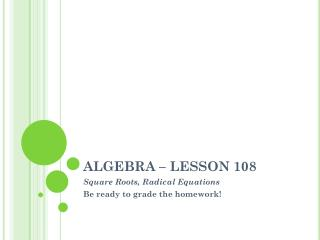DownloadDownload PresentationALGEBRA – LESSON 108

# ALGEBRA – LESSON 108

Download Presentation## ALGEBRA – LESSON 108

- - - - - - - - - - - - - - - - - - - - - - - - - - - E N D - - - - - - - - - - - - - - - - - - - - - - - - - - -
##### Presentation Transcript

2. Grade Scale – 30 possible 29.5 – 98% 22.5 – 75% 29 – 97% 22 – 73% 28.5 – 95% 21.5 – 72% 28 – 93% 21 – 70% 27.5 – 92% 20.5 – 68% 27 – 90% 20 – 67% 26.5 – 88% 19.5 – 65% 26 – 87% 19 – 63% 25.5 – 85% 18.5 – 62% 25 – 83% 18 – 60% 24.5 – 82% 17.5 – 58% 24 – 80% 17 – 57% 23.5 – 78% 16.5 – 55% 23 – 77% 16 – 53%

3. Square Roots Whenever we want to “get rid” of a radical sign, we will square the radical. 4 Steps to remember: 1. Isolate the radical 2. Square both sides 3. Solve 4. Check

4. Square Roots – Just watch Solve: Öx – 2 – 6 = 0 Öx – 2 = 6 (Öx – 2)2 = 62 x – 2 = 36 x = 38 Check: Ö38 – 2 – 6 = 0 Ö36 – 6 = 0 6 – 6 = 0 x = 38

5. Square Roots – Just watch Solve: Öx2 + 9 – 5 = 0 Öx2 + 9 = 5 Ö42 + 9 = 5 (Öx2 + 9)2 = 52 Ö25 = 5 x = 4 x2 + 9 = 25 x2 = 16 Ö(-4)2 + 9 = 5 x2 – 16 = 0 (x + 4)(x – 4)= 0 Ö25 = 5 x = -4 x = -4 or 4

6. Square Roots Solve: Öx – 7 + 3 = -4 Öx – 7 = -7 (Öx – 7)2 = (-7)2 x – 7 = 49 x = 56 Check: Ö56 – 7 + 3 = -4 Ö49 + 3 = -4 7 + 3 = -4 #1 Empty set

7. Square Roots Solve: Öx – 6 + 8 = 2 Öx – 6 = -6 (Öx – 6)2 = (-6)2 x – 6 = 36 x = 42 Check: Ö42 – 6 + 8 = 2 Ö36 + 8 = 2 6 + 8 = 2 #2 Empty set

8. Square Roots Solve: Öe + 25 + 5 = e Ö0 + 25 + 5 = 0 Öe + 25 = e - 5 Ö25 + 5 = 0 (Öe + 25)2= (e - 5)2 Not a solution e + 25 = e2 – 10e + 25 Ö11 + 25 + 5 = 11 Ö36 + 5 = 11 0 = e2 – 11e e = 11 0 = e(e – 11) e = 0 or 11 #3

9. Square Roots Solve: Ö x – 1 – 3 + x = 0 Ö5 – 1 – 3 + 5 = 0 Ö x – 1 = 3 – x Ö4 + 2 = 0 (Ö x – 1)2= (3 – x)2 Not a solution x – 1 = 9 – 6x + x2 Ö2 – 1 – 3 + 2 = 0 Ö1 – 1 = 0 0 = x2 – 7x + 10 x = 2 0 = (x – 5)(x – 2) x = 5 or 2 #4

10. Square Roots Solve: Ö2x – 3 = Öx + 2 Ö2(5) – 3 = Ö5 + 2 Ö7 = Ö7 (Ö2x – 3)2= (Öx + 2)2 x = 5 2x – 3 = x + 2 x – 5 = 0 x = 5 #5

11. Homework: Worksheet

12. Square Roots Solve: 2Öx + 3x - 1 = 0 2 + 3 - 1 = 0 2Öx = -3x + 1 (2Öx )2= (-3x + 1)2 Not a solution 4x = 9x2 – 6x + 1 2(1/3) + 3(1/9) – 1 = 0 2/3 + 1/3 – 1 = 0 0 = 9x2 – 10x + 1 x = 1/9 Use Quadratic Formula x = 1 or 1/9 #4